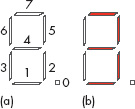Representing and manipulating data in computers

Start this free course now. Just create an account and sign in. Enrol and complete the course for a free statement of participation or digital badge if available.

Free course

# 2.6 Representing true/false quantities

Sometimes a quantity that is to be represented in a computer has only two possible values, either true or false. An example of such a true/false quantity in the kitchen scales is the one that represents whether the scales are to weigh in metric or in imperial measure. The value of this true/false quantity is given by the true/false response to the statement ‘the most recent push of the input button made the measuring system metric’.

## Activity 15 (Exploratory)

The beeper on the timer facility on the scales is either on (sounding) or off (silent). The value of a true/false quantity can be used to represent whether it is on or off. What do you think the statement is that will generate true/false responses for the state of the beeper?

### Discussion

It could be ‘the beeper is on’. Then, if the response is true, the true/ false quantity will be true and the beeper will be sounding; if it is false the true/false quantity will be false and the beeper will be silent.

Note that an alternative is that the statement is ‘the beeper is off’. It is up to the designers to decide which statement is more appropriate in the system and then design the rest of the system to correspond.

True/false quantities such as these are very readily represented by a single bit; all that's needed is to decide whether 1 represents ‘true’ or ‘false’.

Some computers use a single word to hold the value of a true/false quantity, in which case a decision has to be made as to which of the bits in the word is the one that will change depending on whether the value is true or false.

An 8-bit word can, however, hold the values of eight separate true/false quantities if required, making a very compact data representation. This idea could be used for the seven-segment displays in the kitchen scales. Suppose that each individual segment is numbered as shown in Figure 5 (a). Note that most seven-segment displays, including the one used in the kitchen scales, have a decimal point, so I have included one in the figure.Figure 5 (a) Numbering the segments of a seven-segment display; (b) the seven-segment display showing the digit 3

The state of each segment can be represented by the true/false quantity implied by the truthfulness of the statement ‘the segment is lit’. If 1 represents true and 0 represents false then the state of the eight segments when displaying the digit 3 (see Figure 5b) would be:

• segment 0 = 0

• segment 1 = 1

• segment 2 = 1

• segment 3 = 0

• segment 4 = 1

• segment 5 = 1

• segment 6 = 0

• segment 7 = 1

The segments represented by bits 1, 2, 4, 5 and 7 are on; the others are off. This is encoded in very compactly one word as:

 7 6 5 4 3 2 1 0 segment 7 6 5 4 3 2 1 0 bit number 1 0 1 1 0 1 1 0

A true/false quantity like the ones I have been describing in this section is sometimes called a Boolean variable. Hence a Boolean variable is one whose value will be either true or false.

## Activity 16 (Self assessment)

At one time the seven-segment display is showing the digit 2. How would this be represented using the same convention as in the text above?

Using the same convention, the 8-bit word would be 1011 1010 because segments 7, 5, 4, 3 and 1 would be lit.